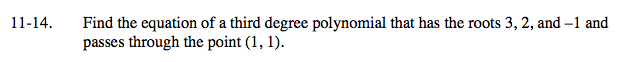Home > A2C > Chapter 11 > Lesson 11.1.1 > Problem11-14

11-14.

Find the equation of a third degree polynomial that has the roots 3, 2, and −1 and passes through the point (1, 1). Homework Help ✎Construct a polynomial equation from the given roots.

f(x) = a(x − 3)(x − 2)(x + 1)

Solve for a by substituting in the given point.